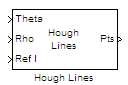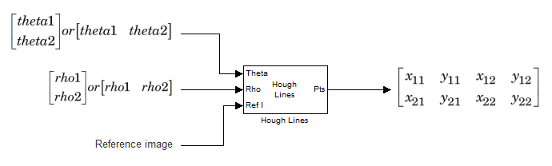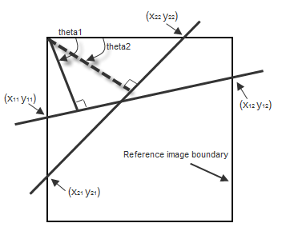# Hough Lines

Find Cartesian coordinates of lines described by rho and theta pairs

• Library:
• Computer Vision Toolbox / Transforms

•## Description

The Hough Lines block finds the points of intersection between the boundary lines of a reference image and a line specified as a rho-theta pair. The block outputs Cartesian coordinates for the points of intersection. The boundary lines indicate the left and right vertical boundaries and the top and bottom horizontal boundaries of the reference image.

If the line specified by a rho-theta pair does not intersect two border lines in the reference image, the block outputs the values, [`0 0 0 0`] for that line. This output value enables the next block in your model to ignore the outputs for that line, as the Hough Lines block generally precedes a block that draws a point or shape at each output intersection.

## Ports

### Input

expand all

Theta value, specified as a scalar or M-element vector. M is the number of specified lines. Each element represents the rotation angle of the corresponding line in radians.

Data Types: `single` | `double` | `int8` | `int16` | `int32` | `uint8` | `uint16` | `uint32` | `fixed point`

Rho value, specified as a scalar or M-element vector. M is the number of specified lines. Each element that represents the distance of the corresponding line from the top-left coordinate origin of the image at the angle specified by the corresponding element of Theta.

Data Types: `single` | `double` | `int8` | `int16` | `int32` | `uint8` | `uint16` | `uint32` | `fixed point`

Reference image, specified as an M-by-N matrix.

Data Types: `single` | `double` | `int8` | `int16` | `int32` | `uint8` | `uint16` | `uint32` | `Boolean` | `fixed point`

### Output

expand all

Intersection points, returned as an M-by-4 matrix of coordinate values, where M is the number of input lines.

For example, consider a Hough Lines block with two-element vectors as the theta and rho inputs, describing two lines.The block outputs a 2-by-4 matrix, in which each row consists of two xy-pairs of coordinates that describe the points at which the corresponding line intersects with the boundary lines of the reference image.

```[x11 y11 x12 y12; ```

```x21 y21 x22 y22]```

This figure shows the line described by the first rho-theta pair intersecting the boundaries of the reference image at [```x11 y11 x12 y12```] and the line described by the second pair intersecting the boundaries at [```x21 y21 x22 y22```].Data Types: `int32`

## Parameters

expand all

You can specify one of these options.

• `Table lookup` — Before the simulation starts, the block computes and stores the trigonometric values required to calculate the intersections of the lines. In this case, the block requires additional memory.

• `Trigonometric function` — The block computes the sine and cosine values required to calculate the intersections of the lines during the simulation.

Specify the spacing of the theta-axis. Value must be greater than 0 and less than `pi/2`.

#### Dependencies

To enable this parameter, set the Sine value computation method parameter to `Table lookup`.

## Block Characteristics

 Data Types `Booleana` | `double` | `fixed pointb, c` | `integerd` | `single` Multidimensional Signals `no` Variable-Size Signals `yes` a This data type is only supported at the input port Ref1.b Generated code will be restricted to MATLAB host computers when you set the FFT implementation parameter to FFTW, or when the transform length is not a power of two.c Input ports Theta and Rho support signed fixed-point data types with word lengths less than or equal to 32, while Ref1 supports signed and unsigned fixed-point data types.d The output port supports 32-bit signed integers only. Input ports Theta and Rho support signed integers, while Ref1 supports signed and unsigned integers.

## Version History

Introduced before R2006a